# chowtest

Chow test for structural change

## Syntax

``h = chowtest(X,y,bp)``
``````[h,pValue,stat,cValue] = chowtest(X,y,bp)``````
``StatTbl = chowtest(Tbl,bp)``
``___ = chowtest(___,Name=Value)``

## Description

Chow tests assess the stability of coefficients β in a multiple linear regression model of the form y = Xβ + ε. `chowtest` splits the data at specified break points. Coefficients are estimated in initial subsamples, then tested for compatibility with data in complementary subsamples.

example

````h = chowtest(X,y,bp)` returns a vector of test decisions `h` from conducting Chow tests on the multiple linear regression model `y` = `X`β + ε at the break points in `bp`. `y` is a vector of response data and `X` is a matrix of predictor data. Each element of `bp` results in a separate test.```

example

``````[h,pValue,stat,cValue] = chowtest(X,y,bp)``` additionally returns vectors of p-values `pValue`, test statistics `stat`, and critical values `cValue` for the tests.```

example

````StatTbl = chowtest(Tbl,bp)` returns the table `StatTbl` containing variables for the test results, statistics, and settings from conducting Chow tests on the variables of the table or timetable `Tbl`. Each row of `StatTbl` contains the results of the corresponding test.The response variable in the regression is the last table variable, and all other variables are the predictor variables. To select a different response variable for the regression, use the `ResponseVariable` name-value argument. To select different predictor variables, use the `PredictorNames` name-value argument.```

example

````___ = chowtest(___,Name=Value)` uses additional options specified by one or more name-value arguments, using any input-argument combination in the previous syntaxes. `chowtest` returns the output-argument combination for the corresponding input arguments. In addition to `bp`, some options control the number of tests to conduct. For example, ```chowtest(Tbl,ResponseVariable="GDP",Test=["breakpoint" "forecast"],Intercept=false)``` conducts two tests for the presence of a structural break in the coefficients of the regression model of `GDP` on all other variables of the table `Tbl` without an intercept term. The first test assesses coefficient equality constraints directly, and the second test assesses forecast performance.```

## Examples

collapse all

Conduct Chow tests to assess whether there are structural changes in the equation for food demand around World War II. Input the predictor series as a matrix and input the response series as a vector.

Load the US food consumption data set `Data_Consumption.mat`, which contains annual measurements from 1927 through 1962 with missing data due to the war in the matrix `Data`.

`load Data_Consumption`

Suppose that you want to develop a model for consumption as determined by food prices and disposable income, and assess its stability through the economic shock through the war.

Plot the series.

```P = Data(:,1); % Food price index I = Data(:,2); % Disposable income index Q = Data(:,3); % Food consumption index figure; plot(dates,[P I Q]) axis tight grid on xlabel("Year") ylabel("Index") legend(["Price" "Income" "Consumption"],Location="southeast")```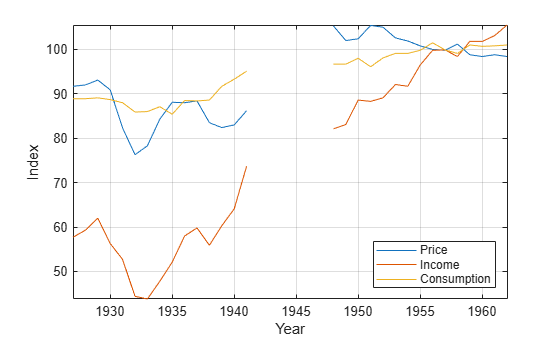Measurements are missing from 1942 through 1947, which correspond to World War II.

Stabilize each series by applying the log transformation.

```LP = log(P); LI = log(I); LQ = log(Q);```

Assume that log consumption is a linear function of the logs of food price and income.

`${\text{LQ}}_{t}={\beta }_{0}+{\beta }_{1}{\text{LI}}_{t}+{\beta }_{2}\text{LP}+{\epsilon }_{t}.$`

${\epsilon }_{t}$ is a Gaussian random variable with mean 0 and standard deviation ${\sigma }^{2}$.

Identify the indices before World War II. Plot log consumption with respect to the logs of food price and income.

```preWarIdx = (dates <= 1941); figure scatter3(LP(preWarIdx),LI(preWarIdx),LQ(preWarIdx),[],"ro"); hold on scatter3(LP(~preWarIdx),LI(~preWarIdx),LQ(~preWarIdx),[],"b*"); legend(["Pre-war observations" "Post-war observations"], ... Location="best") xlabel("Log Price") ylabel("Log Income") zlabel("Log Consumption") % Obtain better view h = gca; h.CameraPosition = [4.3 -12.2 5.3];```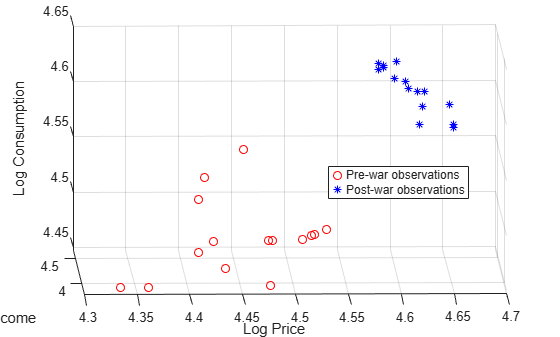Data relationships appear to be affected by the war.

Conduct two break point Chow tests at 5% level of significance. For the first test, set the break point at 1941. Set the break point of the other test at 1948.

```bp = find(preWarIdx,1,"last"); X = [LP LI]; y = LQ; h1941 = chowtest(X,y,bp) ```
```h1941 = logical 1 ```
`h1948 = chowtest(X,y,bp + 1)`
```h1948 = logical 0 ```

`h1941 = 1` indicates that there is enough evidence to reject the null hypothesis that the coefficients are stable when the break points occur before the war. However, `h1948 = 0` indicates that there is not enough evidence to reject coefficient stability if the break point is after the war. This result suggests that the data at 1948 is influential.

Alternatively, you can supply a vector of break points to conduct three Chow tests.

`h = chowtest(X,y,[bp bp+1]);`
```RESULTS SUMMARY *************** Test 1 Sample size: 30 Breakpoint: 15 Test type: breakpoint Coefficients tested: All Statistic: 5.5400 Critical value: 3.0088 P value: 0.0049 Significance level: 0.0500 Decision: Reject coefficient stability *************** Test 2 Sample size: 30 Breakpoint: 16 Test type: breakpoint Coefficients tested: All Statistic: 1.2942 Critical value: 3.0088 P value: 0.2992 Significance level: 0.0500 Decision: Fail to reject coefficient stability ```

By default, `chowtest` displays a summary of the test results for each test when you conduct more than one test.

Load the US food consumption data set `Data_Consumption.mat`. Consider a model for log food consumption as determined by log food prices and log disposable income.

```load Data_Consumption X = log(Data(:,1:2)); y = log(Data(:,3));```

Conduct two break point Chow tests at 5% level of significance. For the first test, set the break point at 1941. Set the break point of the other test at 1948. Return the test decision, $\mathit{p}$-Value, test statistic, and test critical value.

```bp = find(dates <= 1941,1,"last"); [h,pValue,stat,cValue] = chowtest(X,y,bp)```
```h = logical 1 ```
```pValue = 0.0049 ```
```stat = 5.5400 ```
```cValue = 3.0088 ```

`pValue` < 0.01, which suggests that the evidence to reject the null hypothesis that all coefficients in the regression models, determined by the break point at 1941, are equal.

Conduct Chow tests to assess whether there are structural changes in the equation for food demand around World War II, where the time series are variables in a table.

Load the US food consumption data set `Data_Consumption.mat`, which contains annual measurements from 1927 through 1962 with missing data due to the war in the table `DataTable`. Convert the table to a timetable, and remove rows containing missing values.

```load Data_Consumption dates = datetime(dates,12,31); TT = table2timetable(DataTable,RowTimes=dates); TT.Row = []; TT = rmmissing(TT);```

Apply the log transform to all variables in the table.

```LogTT = varfun(@log,TT); LogTT.Properties.VariableNames```
```ans = 1x3 cell {'log_P'} {'log_I'} {'log_Q'} ```

Conduct two break point Chow tests at 5% level of significance. For the first test, set the break point at the end of 1941. Set the break point of the other test at the end of 1948.

```bp1941 = find(LogTT.Time >= datetime(1941,12,31),1); bp1948 = find(LogTT.Time >= datetime(1948,12,31),1); bp = [bp1941 bp1948]; StatTbl = chowtest(LogTT,bp)```
```RESULTS SUMMARY *************** Test 1 Sample size: 30 Breakpoint: 15 Test type: breakpoint Coefficients tested: All Statistic: 5.5400 Critical value: 3.0088 P value: 0.0049 Significance level: 0.0500 Decision: Reject coefficient stability *************** Test 2 Sample size: 30 Breakpoint: 16 Test type: breakpoint Coefficients tested: All Statistic: 1.2942 Critical value: 3.0088 P value: 0.2992 Significance level: 0.0500 Decision: Fail to reject coefficient stability ```
```StatTbl=2×8 table h pValue stat cValue Break Point Alpha Intercept Test _____ _________ ______ ______ ___________ _____ _________ ______________ Test 1 true 0.0049125 5.54 3.0088 15 0.05 true {'breakpoint'} Test 2 false 0.29918 1.2942 3.0088 16 0.05 true {'breakpoint'} ```

`StatTbl` contains the decision statistics and options for each test (row).

By default, `chowtest` selects the last table variable as the response, and selects all other variables as predictors. You can select a different variable by using the `ResponseVariable` name-value argument. You can choose a different set of predictor variables by using the `PredictorVariables` name-value argument.

Apply the Chow test to assess the stability of an explanatory model of US real gross national product (RGNP) using the end of World War II as a break point.

Load the Nelson-Plosser data set `Data_NelsonPlosser.mat`, which contains the table of data `DataTable`.

`load Data_NelsonPlosser`

The time series in the data set contain annual, macroeconomic measurements from 1860 to 1970. For more details, a list of variables, and descriptions, enter `Description` in the command line.

Convert the table to a timetable. Focus the sample to measurements from the end of 1915 through the end of 1970.

```dates = datetime(dates,12,31); span = isbetween(dates,datetime(1915,12,31),datetime(1970,12,31),"closed"); TT = table2timetable(DataTable,RowTimes=dates); TT.Dates = []; TT = TT(span,:);```

Consider a predictive model of the US RGNP `GNPR` given measurements of the industrial production index `IPI`, total employment `E`, and real wages `WR`.

Plot the series in the model.

```prednames = ["IPI" "E" "WR"]; tiledlayout(2,2) for j = ["GNPR" prednames] nexttile plot(TT.Time,TT{:,j}) ylabel(j) end```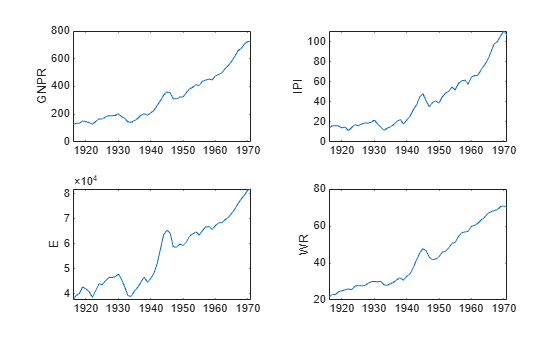To address exponential growth, apply the log transform to the series.

`LogTT = varfun(@log,TT);`

`LogTT` is a timetable containing the transformed variables in `TT`, but with names prepended with `log_`.

Select the index corresponding to the end of World War II, September 2, 1945.

`bp = find(LogTT.Time > datetime(1945,9,2),1);`

Assume that an appropriate multiple regression model to describe real GNP is

`$\mathrm{log}\left({\text{GNPR}}_{t}\right)={\beta }_{0}+{\beta }_{1}\mathrm{log}\left({\text{IPI}}_{t}\right)+{\beta }_{2}\mathrm{log}\left({\text{E}}_{t}\right)+{\beta }_{3}\mathrm{log}\left({\text{WR}}_{t}\right).$`

Conduct a break point test to assess whether all regression coefficients are stable. Use the end of WWII as a break point. Print a test summary to the command line.

```lprednames = "log_" + prednames; StatTbl = chowtest(LogTT,bp,ResponseVariable="log_GNPR", ... PredictorVariables=lprednames,Display="summary")```
```RESULTS SUMMARY *************** Test 1 Sample size: 56 Breakpoint: 31 Test type: breakpoint Coefficients tested: All Statistic: 4.0978 Critical value: 2.5652 P value: 0.0062 Significance level: 0.0500 Decision: Reject coefficient stability ```
```StatTbl=1×8 table h pValue stat cValue Break Point Alpha Intercept Test _____ _________ ______ ______ ___________ _____ _________ ______________ Test 1 true 0.0061633 4.0978 2.5652 31 0.05 true {'breakpoint'} ```

`StatTbl` contains decision statistics and test options for the test. `StatTbl.h = 1` and `StatTbl.pValue < 0.01` indicate string evidence to reject the null hypothesis that the regression coefficients before and after WWII are equivalent.

Conduct a Chow test to assess the stability of a subset of regression coefficients. This example expands on Conduct Chow Test for Structural Change.

Load the US food consumption data set. Convert the table to a timetable, and remove rows containing missing values.

```load Data_Consumption.mat dates = datetime(dates,12,31); TT = table2timetable(DataTable,RowTimes=dates); TT.Row = []; TT = rmmissing(TT);```

Apply the log transformation to each series.

`LogTT = varfun(@log,DataTable);`

Identify the indices before World War II.

`preWarIdx = dates <= datetime(1941,12,31);`

Consider two regression models: one is log consumption onto log food price, and the other is log consumption onto log income. Plot scatter plots and regression lines for both models.

```figure plot(LogTT.log_P(preWarIdx),LogTT.log_Q(preWarIdx),"bo", ... LogTT.log_P(~preWarIdx),LogTT.log_Q(~preWarIdx),"r*"); axis tight grid on lsline xlabel("Log Price") ylabel("Log Consumption") legend("Pre-war observations","Post-war observations")```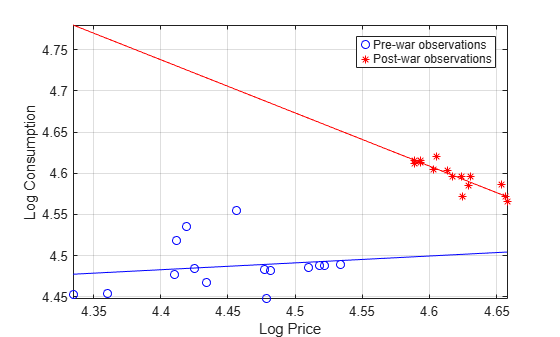```figure plot(LogTT.log_I(preWarIdx),LogTT.log_Q(preWarIdx),"bo", ... LogTT.log_I(~preWarIdx),LogTT.log_Q(~preWarIdx),"r*"); axis tight grid on lsline xlabel("Log Income") ylabel("Log Consumption") legend("Pre-war observations","Post-war observations", ... Location="northwest")```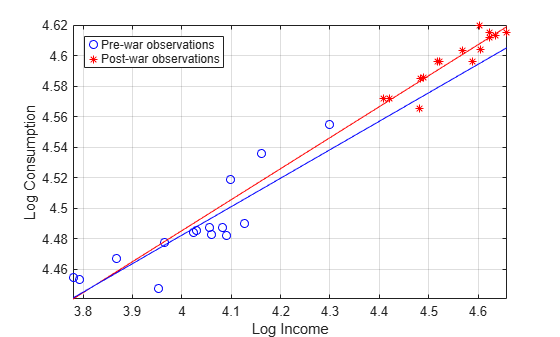A clear break in food price elasticity exists between subsamples before and after the war. However, income elasticity does not appear to have such a break.

Conduct two Chow tests to determine whether there is statistical evidence to reject model continuity for both regression models. Because there are more observations in the complementary subsample than coefficients, conduct a break point test. Consider the elasticities in the test only. That is, specify `false` for the intercept (first coefficient) and `true` for elasticity (second coefficient).

```bp = find(preWarIdx,1,"last"); % Index for 1941 chowtest(LogTT,bp,Coeffs=[false true],Display="summary", ... ResponseVariable="log_Q",PredictorVariables="log_P");```
```RESULTS SUMMARY *************** Test 1 Sample size: 30 Breakpoint: 15 Test type: breakpoint Coefficients tested: 0 1 Statistic: 7.3947 Critical value: 4.2252 P value: 0.0115 Significance level: 0.0500 Decision: Reject coefficient stability ```
```chowtest(LogTT,bp,Coeffs=[false true],Display="summary", ... ResponseVariable="log_Q",PredictorVariables="log_I");```
```RESULTS SUMMARY *************** Test 1 Sample size: 30 Breakpoint: 15 Test type: breakpoint Coefficients tested: 0 1 Statistic: 0.1289 Critical value: 4.2252 P value: 0.7225 Significance level: 0.0500 Decision: Fail to reject coefficient stability ```

The first test rejects the null hypothesis that price elasticities are equivalent across subsamples at 5% level of significance. The second test fails to reject the null hypothesis that income elasticities are equivalent across subsamples.

Consider a regression model of log consumption onto the logs of price and income. Conduct two break point tests: one that compares price elasticity across subsamples only, and another that compares income elasticity only.

```Coeffs = [false true false; false false true]; chowtest(LogTT,bp,Coeffs=Coeffs,Display="summary", ... ResponseVariable="log_Q",PredictorVariables=["log_P" "log_I"]);```
```RESULTS SUMMARY *************** Test 1 Sample size: 30 Breakpoint: 15 Test type: breakpoint Coefficients tested: 0 1 0 Statistic: 0.0001 Critical value: 4.2597 P value: 0.9920 Significance level: 0.0500 Decision: Fail to reject coefficient stability *************** Test 2 Sample size: 30 Breakpoint: 15 Test type: breakpoint Coefficients tested: 0 0 1 Statistic: 2.8151 Critical value: 4.2597 P value: 0.1064 Significance level: 0.0500 Decision: Fail to reject coefficient stability ```

For both tests, there is not enough evidence to reject model stability at 5% level.

Simulate data for a linear model including a structural break in the intercept and one of the predictor coefficients. Then, choose specific coefficients to test for equality across a break point using the Chow test. Adjust parameters to assess the sensitivity of the Chow test.

Specify four predictors, 50 observations, and a break point at period 44 for the simulated linear model.

```numPreds = 4; numObs = 50; bp = 44; rng(1); % For reproducibility```

Form the predictor data by specifying means for the predictors, and then adding random, standard Gaussian noise to each of the means.

```mu = [0 1 2 3]; X = repmat(mu,numObs,1) + randn(numObs,numPreds);```

Include a column of ones to the predictor data.

`X = [ones(numObs,1) X];`

Specify the true values of the regression coefficients and that the intercept and the coefficient of the second predictor jump by 10%.

```beta1 = [1 2 3 4 5]'; % Initial subsample coefficients beta2 = beta1 + [beta1(1)*0.1 0 beta1(3)*0.1 0 0 ]'; % Complementary subsample coefficients X1 = X(1:bp,:); % Initial subsample predictors X2 = X(bp+1:end,:); % Complementary subsample predictors```

Specify a 2-by-5 logical matrix that indicates to test first the intercept and second regression coefficient, and then test all other coefficients.

```test1 = [true false true false false]; Coeffs = [test1; ~test1]```
```Coeffs = 2x5 logical array 1 0 1 0 0 0 1 0 1 1 ```

The null hypothesis for the first test (`Coeffs(1,:)`) is equality of the intercepts and the coefficients of the second predictor across subsamples. The null hypothesis for the second test (`Coeffs(2,:)`) is equality of the first, third, and fourth predictors across subsamples.

Simulate data for the linear model

`$\text{y}=\left[\begin{array}{cc}X1& 0\\ 0& X2\end{array}\right]\left[\begin{array}{c}\text{beta1}\\ \text{beta2}\end{array}\right]+\text{innov}.$`

Create `innov` as a vector of random Gaussian variates with mean zero and standard deviation 0.2.

```sigma = 0.2; innov = sigma*randn(numObs,1); y = [X1 zeros(bp,size(X2,2)); ... zeros(numObs-bp,size(X1,2)) X2]*[beta1; beta2]+innov;```

Conduct the two break point tests indicated in `Coeffs`. Because there is an intercept in the predictor matrix `X` already, specify to suppress its inclusion in the linear model that `chowtest` fits.

```chowtest(X,y,bp,Intercept=false,Coeffs=Coeffs, ... Display="summary");```
```RESULTS SUMMARY *************** Test 1 Sample size: 50 Breakpoint: 44 Test type: breakpoint Coefficients tested: 1 0 1 0 0 Statistic: 5.7102 Critical value: 3.2317 P value: 0.0066 Significance level: 0.0500 Decision: Reject coefficient stability *************** Test 2 Sample size: 50 Breakpoint: 44 Test type: breakpoint Coefficients tested: 0 1 0 1 1 Statistic: 0.2497 Critical value: 2.8387 P value: 0.8611 Significance level: 0.0500 Decision: Fail to reject coefficient stability ```

At the default significance level:

• The Chow test correctly rejects the null hypothesis that no structural breaks exist at period `bp` for the intercept and the second coefficient.

• The Chow test correctly failed to reject the null hypothesis for the other coefficients.

Compare the break point test results to the results of the forecast test.

```chowtest(X,y,bp,Intercept=false,Coeffs=Coeffs, ... Test="forecast",Display="summary");```
```RESULTS SUMMARY *************** Test 1 Sample size: 50 Breakpoint: 44 Test type: forecast Coefficients tested: 1 0 1 0 0 Statistic: 3.7637 Critical value: 2.8451 P value: 0.0182 Significance level: 0.0500 Decision: Reject coefficient stability *************** Test 2 Sample size: 50 Breakpoint: 44 Test type: forecast Coefficients tested: 0 1 0 1 1 Statistic: 0.2135 Critical value: 2.6123 P value: 0.9293 Significance level: 0.0500 Decision: Fail to reject coefficient stability ```

In this case, the inferences from the tests are equivalent to those for the break point test.

## Input Arguments

collapse all

Predictor data X for the multiple linear regression model, specified as a `numObs`-by-`numPreds` numeric matrix.

Each row represents one of the `numObs` observations and each column represents one of the `numPreds` predictor variables.

Data Types: `double`

Response data y for the multiple linear regression model, specified as a `numObs`-by-1 numeric vector. Rows of `y` and `X` correspond.

Data Types: `double`

Combined predictor and response data for the multiple linear regression model, specified as a table or timetable with `numObs` rows. Each row of `Tbl` is an observation.

The test regresses the response variable, which is the last variable in `Tbl`, on the predictor variables, which are all other variables in `Tbl`. To select a different response variable for the regression, use the `ResponseVariable` name-value argument. To select different predictor variables, use the `PredictorNames` name-value argument to select `numPreds` predictors.

Break points for the tests, specified as a positive integer or a vector of positive integers.

Each break point is an index of a specific observation (row) in the data. The element `bp(j)` specifies to split the data into the initial and complementary samples indexed by `1:bp(j)` and `(bp(j) + 1):numObs`, respectively.

Data Types: `double`

Note

• `NaN`s in `X`, `y`, or `Tbl` indicate missing values, and `chowtest` removes observations containing at least one `NaN`. That is, to remove `NaN`s in `X` or `y`, `chowtest` merges the variables ```[X y]```, and then it uses list-wise deletion to remove any row that contains at least one `NaN`. `chowtest` also removes any row of `Tbl` containing at least one `NaN`. Removing `NaN`s in the data reduces the sample size and can create irregular time series.

• If `bp` is a scalar, then the number of tests, `numTests`, is the common dimension of values in name-value argument. In this case, `chowtest` uses the same `bp` in each test. Otherwise, the length of `bp` determines `numTests`, and `chowtest` runs separate tests for each value in `bp`.

### Name-Value Arguments

Specify optional pairs of arguments as `Name1=Value1,...,NameN=ValueN`, where `Name` is the argument name and `Value` is the corresponding value. Name-value arguments must appear after other arguments, but the order of the pairs does not matter.

Before R2021a, use commas to separate each name and value, and enclose `Name` in quotes.

Example: ```chowtest(Tbl,DataVariable="GDP",Test=["breakpoint" "forecast"],Intercept=true)``` conducts two tests for the presence of a structural break in the coefficients, including an intercept, of the regression model of `GDP` on all other variables of the table `Tbl`. The first test assesses coefficient equality constraints directly, and the second test assesses forecast performance.

Flag to include an intercept when `chowtest` fits the regression model, specified as a value in this table or a length `numTests` vector of such values.

ValueDescription
`true``chowtest` includes an intercept when fitting the regression model. `numCoeffs` = `numPreds` + 1.
`false``chowtest` does not include an intercept when fitting the regression model. `numCoeffs` = `numPreds`.

`chowtest` conducts a separate test for each value in `Intercept`.

Example: `Intercept=false` excludes an intercept from the model for each test.

Data Types: `logical`

Chow test type, specified as a test name, or a string vector or cell vector of test names. This table contains the supported test names.

Test NameDescription
`"breakpoint"` (default)
• `chowtest` directly assesses coefficient equality constraints using an F statistic.

• Both subsamples must have more than `numCoeffs` observations.

`"forecast"`
• `chowtest` assesses forecast performance using a modified F statistic.

• The initial subsample must have more than `numCoeffs` observations.

For details on the value of `numCoeffs`, see the `Intercept` and `Coeffs` name-value arguments.

`chowtest` conducts a separate test for each test name in `Test`.

Example: `Test=["breakpoint" "forecast"]` conducts two Chow tests. The first test directly assesses coefficient equality constraints, and the second test assess forecast performance.

Data Types: `char` | `string` | `cell`

Flags indicating which elements of β to test for equality, specified as a logical vector or array. Vector values must be of length `numCoeffs`. Array values must be of size `numTests`-by-`numCoeffs`.

If the value of `Intercept` contains mixed logical values:

• `numCoeffs` is `numPreds` + 1

• `chowtest` ignores values in the first column of `Coeffs` for models without an intercept.

For example, suppose the regression model has three predictors (`numPreds` is 3) in a linear model, and you want to conduct two Chow tests (`numTests` is 2). Each test includes all regression parameters in the linear model. Also, you want `chowtest` to fit an intercept in the linear model for the first test only. Therefore, `Intercept` must be the logical array ```[1 0]```. Because there is at least one model for which `chowtest` fits an intercept, `Coeffs` must be a 2-by-4 logical array (`numTests` is 2 and `numCoeffs` is `numPreds` + 1). The elements of `Coeffs(:,1)` correspond to whether to test the intercept irrespective of its presence in the model. Therefore, one way to specify `Coeffs` is `true(2,4)`. For the second test, `chowtest` does not fit an intercept, and so it ignores the value `true` in `Coeffs(2,1)`. Because `chowtest` ignores `Coeffs(2,1)`, `Coeffs` = `[true(1,4); false true(1,3)]` yields the same result.

The default is `true(numTests,numCoeffs)`, which tests all of β for all tests.

Example: `Coeffs=[false true true; true true true]` conducts two Chow tests on a model containing three coefficients. The first test assesses the second and third coefficients, and the second test assesses all coefficients.

Nominal significance level for the hypothesis test, specified as a numeric scalar in the interval (0,1) or a numeric vector of such values.

`chowtest` conducts a separate test for each value in `Alpha`.

Example: `Alpha=[0.01 0.05]` uses a level of significance of `0.01` for the first test, and then uses a level of significance of `0.05` for the second test.

Data Types: `double`

Flag for a command window display of results, specified as a value in this table.

ValueDescriptionDefault Value When
`"off"``chowtest` does not display results in the command window.`numTests` = 1
`"summary"`For each test, `chowtest` displays results in the command window.`numTests` > 1

The value of `Display` applies to all tests.

Example: `Display="off"`

Data Types: `char` | `string`

Variable in `Tbl` to use for response, specified as a string vector or cell vector of character vectors containing variable names in `Tbl.Properties.VariableNames`, or an integer or logical vector representing the indices of names. The selected variables must be numeric.

`chowtest` uses the same specified response variable for all tests.

Example: `ResponseVariable="GDP"`

Example: `ResponseVariable=[true false false false]` or `ResponseVariable=1` selects the first table variable as the response.

Data Types: `double` | `logical` | `char` | `cell` | `string`

Variables in `Tbl` to use for the predictors, specified as a string vector or cell vector of character vectors containing variable names in `Tbl.Properties.VariableNames`, or an integer or logical vector representing the indices of names. The selected variables must be numeric.

`chowtest` uses the same specified predictors for all tests.

By default, `chowtest` uses all variables in `Tbl` that are not specified by the `ResponseVariable` name-value argument.

Example: `PredictorVariables=["UN" "CPI"]`

Example: `PredictorVariables=[false true true false]` or `DataVariables=[2 3]` selects the second and third table variables.

Data Types: `double` | `logical` | `char` | `cell` | `string`

Note

• When `chowtest` conducts multiple tests, the function applies all single settings (scalars or character vectors) to each test.

• All vector-valued specifications that control the number of tests must have equal length. Vector values and `Coeffs` arrays must share a common dimension, equal to `numTests`.

• If you specify `X` and `y`, and `bp`, `Intercept`, `Test`, or `Alpha` are row vectors, `chowtest` returns row vectors.

## Output Arguments

collapse all

Test rejection decisions, returned as a logical scalar or vector with length equal to the number of tests `numTests`. `chowtest` returns `h` when you supply the inputs `X` and `y`.

The Chow test has the following hypotheses are:

• H0: Regression coefficients β, selected by the `Coeffs` name-value argument, are identical across subsamples.

• H1: At least one regression coefficient in β, selected by the `Coeffs` name-value argument, exhibits significant change across subsamples.

Elements of `h` have the following values and meanings.

• Values of `1` indicate rejection of the null hypothesis that the regression coefficients β, selected by `Coeffs`, are identical across subsamples model, in favor of the alternative hypothesis.

• Values of `0` indicate failure to reject the null hypothesis.

Test statistic p-values, returned as a numeric scalar or vector with length equal to the number of tests `numTests`. `chowtest` returns `pValue` when you supply the inputs `X` and `y`.

Test statistics, returned as a numeric scalar or vector with length equal to the number of tests `numTests`. `chowtest` returns `stat` when you supply the inputs `X` and `y`. For details, see Chow Tests.

Critical values, returned as a numeric scalar or vector with length equal to the number of tests `numTests`. `chowtest` returns `cValue` when you supply the inputs `X` and `y`.

`Alpha` determines the critical values.

Test summary, returned as a table with variables for the outputs `h`, `pValue`, `stat`, and `cValue`, and with a row for each test. `chowtest` returns `StatTbl` when you supply the input `Tbl`.

`StatTbl` contains variables for the break point `Break Point` and test settings specified by `Alpha`, `Intercept`, and `Test`.

collapse all

### Chow Tests

Chow tests assess the stability of the coefficients β in a multiple linear regression model of the form y = Xβ + ε. `chowtest` supports the two variations of the Chow test introduced in : the break point and forecast tests.

The break point test is a standard F test from the analysis of covariance. The forecast test makes use of the standard theory of prediction intervals. Chow’s contribution is to place both tests within the general linear hypothesis framework, and then to develop appropriate test statistics for testing subsets of coefficients (see `Coeffs`). For test-statistic formulae, see .

## Tips

• Chow tests assume continuity of the innovations variance across structural changes. Heteroscedasticity can distort the size and power of the test. Therefore, verify the innovations-variance-continuity assumption holds before using the test results for inference.

• If both subsamples contain more than `numCoeffs` observations, you can conduct a forecast test instead of a break point test (see `Test`). However, the forecast test might have lower power relative to the break point test . Nevertheless, Wilson (1978) suggests conducting the forecast test in the presence of unknown specification errors .

• You can apply the forecast test to cases where both subsamples have size greater than `numCoeffs`, where you would typically apply a breakpoint test. In such cases, the forecast test might have significantly reduced power relative to a break point test . Nevertheless, Wilson (1978) suggests use of the forecast test in the presence of unknown specification errors .

• The forecast test is based on the unbiased predictions, with zero mean error, that result from stable coefficients. However, zero mean forecast error does not, in general, guarantee coefficient stability. Therefore, forecast tests are most effective in checking for structural breaks, rather than model continuity .

• To obtain diagnostic statistics for each subsample, such as regression coefficient estimates, their standard errors, error sums of squares, and so on, pass the appropriate data to `fitlm`. For details on working with `LinearModel` model objects, see Multiple Linear Regression.

 Chow, G. C. "Tests of Equality Between Sets of Coefficients in Two Linear Regressions." Econometrica. Vol. 28, 1960, pp. 591–605.

 Fisher, F. M. "Tests of Equality Between Sets of Coefficients in Two Linear Regressions: An Expository Note." Econometrica. Vol. 38, 1970, pp. 361–66.

 Rea, J. D. "Indeterminacy of the Chow Test When the Number of Observations is Insufficient." Econometrica. Vol. 46, 1978, p. 229.

 Wilson, A. L. "When is the Chow Test UMP?" The American Statistician. Vol. 32, 1978, pp. 66–68.